Do you like the article?
Share it with others -
post a link to it!

Use new possibilities of MetaTrader 5

# MTF indicators as the technical analysis tool

9 May 2019, 10:48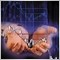1
4 658

### Introduction

Most of traders agree that the current market state analysis starts with the evaluation of higher chart timeframes. The analysis is performed downwards to lower timeframes until the one, at which deals are performed. This analysis method seems to be a mandatory part of professional approach for successful trading. To perform multi-timeframe analysis, users often have to open several windows or switch between chart periods if the same set of instruments is used. Thus the preliminary analysis is performed.

What to do next? Does one have to ignore older timeframes or keep switching between windows and periods? When the working timeframe is Н1 or above, the user has time for careful evaluation of the general situation. However, there is no time for checking required information form higher periods when working on the M1-M15 timeframes. While this information can sometimes be vital. Moreover, the data should be available right here and now, not on another tab or anywhere else. This is especially important for MTF strategies based on the simultaneous evaluation of different TFs, such as the Wolfe Waves or the Elder's Three Screens.

Thus traders using these strategies are often in the state of mental strain. When performing trades on lower TFs, one can miss profitable signals on higher periods. This may lead to messy decisions, early closing of positions or missing of market reversal points. The consequences can be very upsetting. In these situations, the user can only rely on their experience or information accessing speed.

The solution to this problem is an indicator which receives information from different timeframes or multiple trading symbols and displays the comprehensive data on the screen, so that the user can efficiently assess the market state. In addition to basic information, these indicators can display the real state of the market trend and recommend further trading activities.

### Algorithm features

The main difference from the classical indicators is that MTF indicators can process generalized information from all time intervals or financial instruments and then pass data to the current chart. Any indicator type (oscillator, trend, volume, etc.) or a combination of them can operate on multiple timeframes. They are calculated according to their basic algorithm and pass information in accordance with the time interval specified in the settings.

No special setup is needed for MTF versions as compared to classical ones, except for the additional parameter (or group of parameters) to specify time intervals or list of symbols to use data from. The result can be output in the main chart window or separately, as well as be displayed as a signal while combining groups based on the instrument type.

### Classification of multi-timeframe indicators

These indicators are presented in all standard classes, while most of them are of the complex type, i.e. combine calculations and graphical elements. The indicator groups are shown below:

1. Informational: such indicators displays data and additional information without signals or graphics. A classic example is the MultiTimeFrame indicator. It shows candlestick closing time on each timeframe, Ask and Bid for the selected currency pairs, the candlestick state (UP, DOWN, DOJI) and the volume. The indicator screen is filled with a large amount of useful information, which however is hard to use in trading and can only be viewed.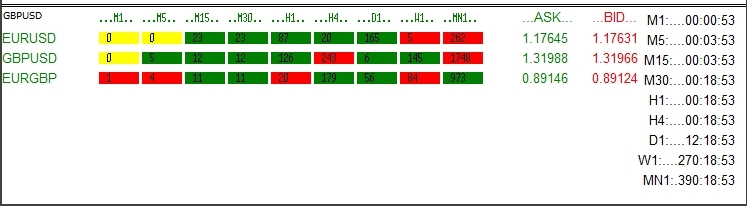Fig. 1. Information indicators

This group also features independent tools which can be used to make trading decisions. They display the analysis results of several standard indicators without the need to install such indicators on a chart (the calculation is performed automatically), as well as generate trade recommendations.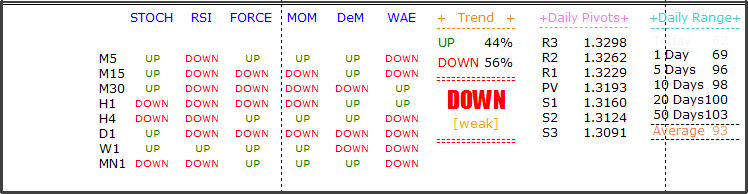Fig. 2. Signals of information indicators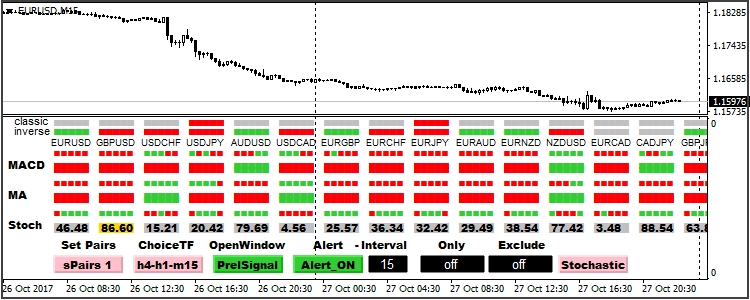Fig. 3. Signals of information indicators

2. Graphical indicators show graphics of the same tool on different TFs. Here is the standard Envelope MA(13) from three different timeframes.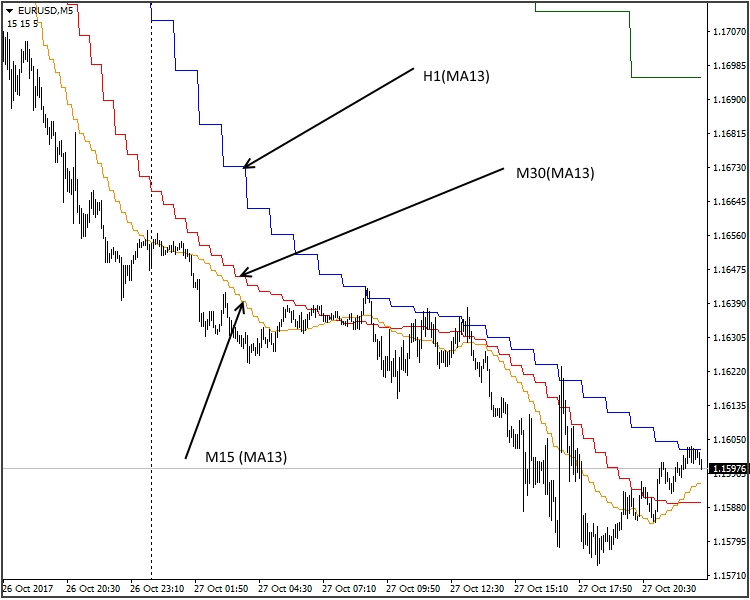Fig. 4. Graphical indicators

Another type of graphical construction is the group of charts with different calculation period. This approach is based on pure mathematics. I.e. the Stochastic indicator (5.3.3) on M5 will have the parameters of (15.3.9) from M15 and (30.3.18) from M30.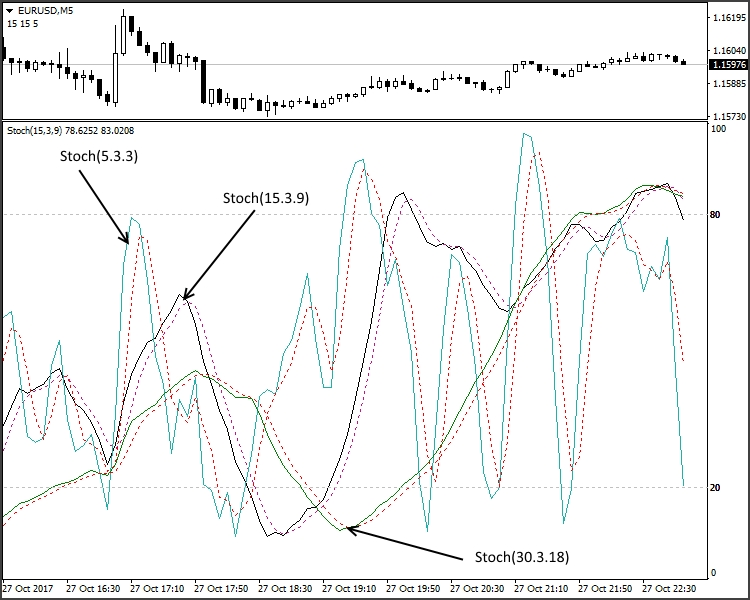Fig. 5. Graphical indicators with different calculation periods

The above version can be referred to the MTF class with some reservation. It is not always possible to implement this approach, while the disadvantages of this solution can be so significant that the use of it would be inappropriate. We will dwell on cases where the method is applicable as well as on its advantages and disadvantages later.

A separate group consists of the so called signal indicators. In order to avoid too many graphical constructions on the working area, the indicator forms signal lines or graphical blocks reflecting the trend direction or other parameters. See the standard MTF_Coral indicator with the above MTF solution: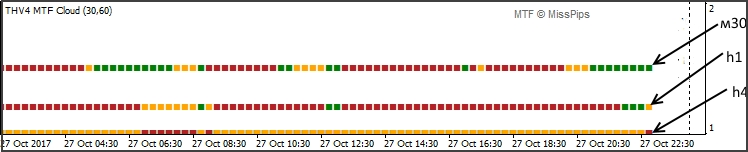Fig. 6. Signal indicators

Another group can be called "Window in a window". Charts of different timeframes or indicators are shown within the main chart window.Fig. 7. "Window in window" indicators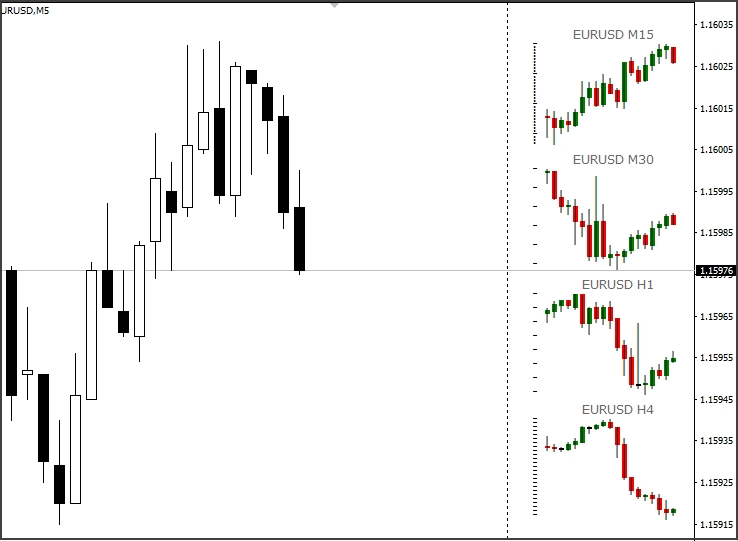Fig. 7.1. "Window in window" indicator

Another example of All_Woodies CCI.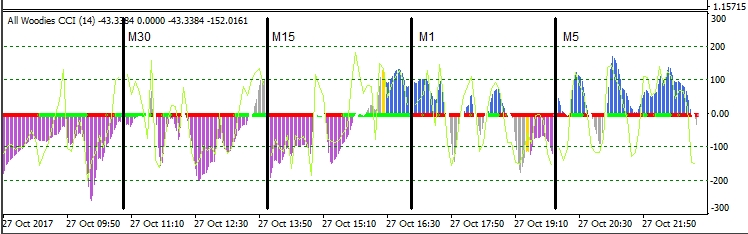Fig. 7.1. "Window in window" indicator All_Woodies CCI

A separate group includes MTF volatility indicators. These include MTF Candles.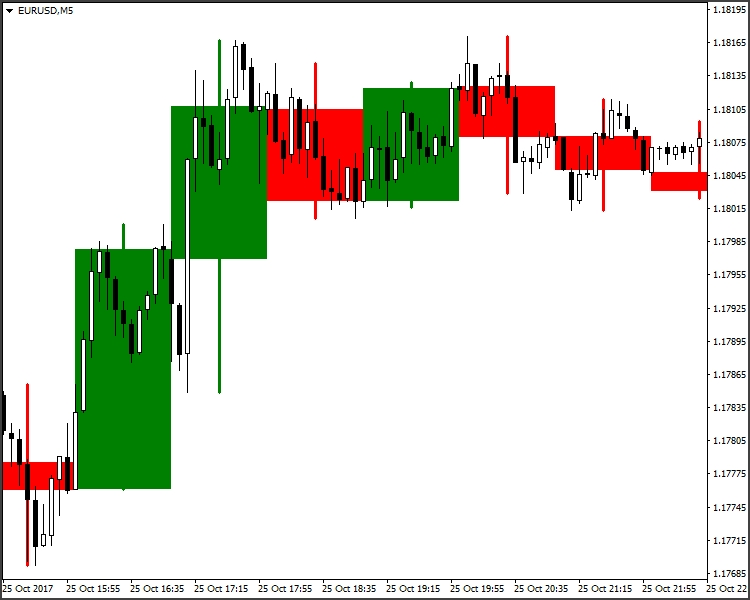Fig. 8. Volatility indicator MTF Candles

### Implementation methods

We have considered the main types of MTF indicators. Now let us view simple examples which show the main methods for linear implementation. We will also analyze the specific features of each solution.

Multiperiod indicators.

Let us consider the MA indicator and try to solve the following task: Create an indicator version with a changing calculation period to show three different TFs.

Let us set the main parameters and our variables:

```//---- indicator settings
#property indicator_chart_window
#property indicator_buffers 3
#property indicator_plots   3
#property indicator_type1   DRAW_LINE
#property indicator_type2   DRAW_LINE
#property indicator_type3   DRAW_LINE
#property indicator_color1  Blue
#property indicator_color2  Red
#property indicator_color3  Lime
#property indicator_width1  1
#property indicator_width2  1
#property indicator_width3  1
//---- input parameters
input ENUM_TIMEFRAMES    tf1             = 1;              // Time Frame (1)
input ENUM_TIMEFRAMES    tf2             = 5;              // Time Frame (2)
input ENUM_TIMEFRAMES    tf3             = 15;             // Time Frame (3)
input int                maPeriod        = 13;             // MA period
input int                Shift           = 0;              // Shift
input ENUM_MA_METHOD     InpMAMethod     = MODE_SMA;      // Moving average method
input ENUM_APPLIED_PRICE InpAppliedPrice = PRICE_CLOSE;   // Applied price
//---- indicator buffers
double ExtBuf1[];
double ExtBuf2[];
double ExtBuf3[];
//---- handles for moving averages
int    ExtHandle1;
int    ExtHandle2;
int    ExtHandle3;
//--- bars minimum for calculation
int    ExtBarsMinimum;
//---
int period1=0;
int period2=0;
int period3=0;
```

Now implement array data with the condition that the TF it is attached to is <= than those specified in variables.

```void OnInit()
{
int timeframe;
//---- indicator buffers mapping
SetIndexBuffer(0,ExtBuf1,INDICATOR_DATA);
SetIndexBuffer(1,ExtBuf2,INDICATOR_DATA);
SetIndexBuffer(2,ExtBuf3,INDICATOR_DATA);
//---
timeframe =_Period;
//---
if(tf1>=timeframe)
{
period1=maPeriod*(int)MathFloor(tf1/timeframe);
PlotIndexSetInteger(0,PLOT_DRAW_BEGIN,period1-1);             //sets first bar from what index will be drawn
PlotIndexSetInteger(0,PLOT_SHIFT,Shift);                      //line shifts when drawing
PlotIndexSetString(0,PLOT_LABEL,"MA("+string(period1)+")");   //name for DataWindow
ExtHandle1=iMA(NULL,0,period1,0,InpMAMethod,InpAppliedPrice); //get MA's handles
}
//----
if(tf2>=timeframe)
{
period2=maPeriod*(int)MathFloor(tf2/timeframe);
PlotIndexSetInteger(1,PLOT_DRAW_BEGIN,period2-1);             //sets first bar from what index will be drawn
PlotIndexSetInteger(1,PLOT_SHIFT,Shift);                      //line shifts when drawing
PlotIndexSetString(1,PLOT_LABEL,"MA("+string(period2)+")");   //name for DataWindow
ExtHandle2=iMA(NULL,0,period2,0,InpMAMethod,InpAppliedPrice); //get MA's handles
}
//----
if(tf3>=timeframe)
{
period3=maPeriod*(int)MathFloor(tf3/timeframe);
PlotIndexSetInteger(2,PLOT_DRAW_BEGIN,period3-1);             //sets first bar from what index will be drawn
PlotIndexSetInteger(2,PLOT_SHIFT,Shift);                      //line shifts when drawing
PlotIndexSetString(2,PLOT_LABEL,"MA("+string(period3)+")");   //name for DataWindow
ExtHandle3=iMA(NULL,0,period3,0,InpMAMethod,InpAppliedPrice); //get MA's handles
}
//--- set accuracy
IndicatorSetInteger(INDICATOR_DIGITS,_Digits);
//--- bars minimum for calculation
int per=MathMax(period3,MathMax(period1,period2));
ExtBarsMinimum=per+Shift;
//--- initialization done
}
```

The main loop to check initialization and calculation:

```int OnCalculate(const int rates_total,
const int prev_calculated,
const datetime &time[],
const double &open[],
const double &high[],
const double &low[],
const double &close[],
const long &tick_volume[],
const long &volume[],
const int &spread[])
{
//--- check for rates total
if(rates_total<ExtBarsMinimum)
return(0); // not enough bars for calculation
//--- not all data may be calculated
int calculated=BarsCalculated(ExtHandle1);
if(calculated<rates_total&&period1!=0)
{
Print("Not all data of ExtHandle1 is calculated (",calculated,"bars ). Error",GetLastError());
return(0);
}
calculated=BarsCalculated(ExtHandle2);
if(calculated<rates_total&&period2!=0)
{
Print("Not all data of ExtHandle2 is calculated (",calculated,"bars ). Error",GetLastError());
return(0);
}
calculated=BarsCalculated(ExtHandle3);
if(calculated<rates_total&&period3!=0)
{
Print("Not all data of ExtHandle3 is calculated (",calculated,"bars ). Error",GetLastError());
return(0);
}
//--- we can copy not all data
int to_copy;
if(prev_calculated>rates_total || prev_calculated<0) to_copy=rates_total;
else
{
to_copy=rates_total-prev_calculated;
if(prev_calculated>0) to_copy++;
}
//---- get ma buffers
if(IsStopped()) return(0); //Checking for stop flag
if(period1!=0)
if(CopyBuffer(ExtHandle1,0,0,to_copy,ExtBuf1)<=0)
{
Print("getting ExtHandle1 is failed! Error",GetLastError());
return(0);
}
if(IsStopped()) return(0); //Checking for stop flag
if(period2!=0)
if(CopyBuffer(ExtHandle2,0,0,to_copy,ExtBuf2)<=0)
{
Print("getting ExtHandle2 is failed! Error",GetLastError());
return(0);
}
if(IsStopped()) return(0); //Checking for stop flag
if(period3!=0)
if(CopyBuffer(ExtHandle3,0,0,to_copy,ExtBuf3)<=0)
{
Print("getting ExtHandle3 is failed! Error",GetLastError());
return(0);
}
//--- return value of prev_calculated for next call
return(rates_total);
}
//+------------------------------------------------------------------+
```

Let's look at the result.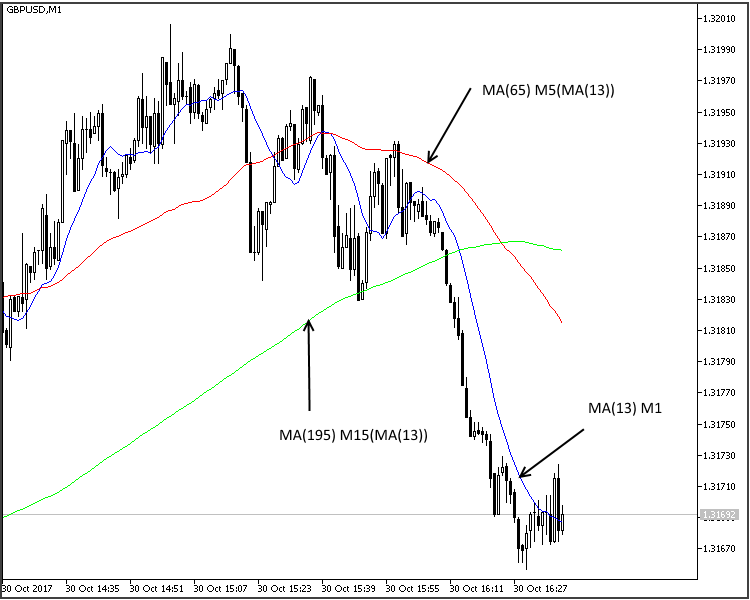Fig. 9. Implementation of an MTF indicator

We have considered the example of using the same indicator in different timeframes using the calculation period increase. With a slight alteration, we can use while providing the possibility to set individual periods for each line. This method may seem inefficient. It is very easy to calculate the period and apply multiple indicators simultaneously. However, in some cases this method is the optimal one despite all its disadvantages. One of them is the necessity to simultaneously observe two (three) non-normalized oscillators in one window. Due to the amplitude range, these oscillators are shifted relative to the center line and thus their interpretation becomes difficult. This method eliminates the above disadvantage.

### Multi-timeframe indicators

Instead of the well known MQL4 functions iClose(), iHigh(), iLow(), iOpen(), iTime(), iVolume(), MQL5 provides functions CopyTime(), CopyClose(), CopyHigh(), CopyLow(), CopyOpen(), CopyTime(), CopyVolume(), while functions iCustom, iMA, iCCI, iMACD and others are implemented via CopyBuffer(). Each of them has its advantages and disadvantages. In our case we will only consider MQL5. For our indicator we need the entire list of timeframes from M1 to MN1, i.e. 26 versions. Further, if you need to use several trade symbols or instruments, this number increases many times. In most cases there is no need to copy the entire history. For most of information indicators the number of bars is limited by two. Therefore, in order to prevent the code from becoming too lengthy, it is better to write these commands as separate functions and call them repeatedly.

For the timeseries function CopyClose(), the function will be as follows:

```//+------------------------------------------------------------------+
double _iClose(string symbol,int tf,int index)
{
if(index < 0) return(-1);

double buf[];
ENUM_TIMEFRAMES timeframe=TFMigrate(tf);
if(CopyClose(symbol,timeframe, index, 1, buf)>0)
return(buf);
else return(-1);
}
//+------------------------------------------------------------------+
```

For WPR call:

```//+------------------------------------------------------------------+
double _iWPR(string symbol,
int tf,
int period,
int shift)
{
ENUM_TIMEFRAMES timeframe=TFMigrate(tf);
int handle=iWPR(symbol,timeframe,period);
if(handle<0)
{
Print("The iWPR object not created: Error ",GetLastError());
return(-1);
}
else
return(_CopyBuffer(handle,shift));
}
//+------------------------------------------------------------------+
double _CopyBuffer(int handle,int shift)
{
double buf[];
if(CopyBuffer(handle,0,shift,1,buf)>0)
return(buf);

return(EMPTY_VALUE);
}
//+------------------------------------------------------------------+
```

If there are several lines, _CopyBuffer can be written as follows:

```//+------------------------------------------------------------------+
double _CopyBuffer(int handle,int index,int shift)
{
double buf[];
switch(index)
{
case 0: if(CopyBuffer(handle,0,shift,1,buf)>0)
return(buf); break;
case 1: if(CopyBuffer(handle,1,shift,1,buf)>0)
return(buf); break;
case 2: if(CopyBuffer(handle,2,shift,1,buf)>0)
return(buf); break;
case 3: if(CopyBuffer(handle,3,shift,1,buf)>0)
return(buf); break;
case 4: if(CopyBuffer(handle,4,shift,1,buf)>0)
return(buf); break;
default: break;
}
return(EMPTY_VALUE);
}
//+------------------------------------------------------------------+
```

while the _iWPR function will change the line

`return(_CopyBuffer(handle,shift)`

to

`return(_CopyBuffer(handle,0,shift)`

in both cases TFMigrate() will look as follows:

```//+------------------------------------------------------------------+
ENUM_TIMEFRAMES TFMigrate(int tf)
{
switch(tf)
{
case 0: return(PERIOD_CURRENT);
case 1: return(PERIOD_M1);
case 5: return(PERIOD_M5);
case 15: return(PERIOD_M15);
case 30: return(PERIOD_M30);
case 60: return(PERIOD_H1);
case 240: return(PERIOD_H4);
case 1440: return(PERIOD_D1);
case 10080: return(PERIOD_W1);
case 43200: return(PERIOD_MN1);

case 2: return(PERIOD_M2);
case 3: return(PERIOD_M3);
case 4: return(PERIOD_M4);
case 6: return(PERIOD_M6);
case 10: return(PERIOD_M10);
case 12: return(PERIOD_M12);
case 20: return(PERIOD_M20);
case 16385: return(PERIOD_H1);
case 16386: return(PERIOD_H2);
case 16387: return(PERIOD_H3);
case 16388: return(PERIOD_H4);
case 16390: return(PERIOD_H6);
case 16392: return(PERIOD_H8);
case 16396: return(PERIOD_H12);
case 16408: return(PERIOD_D1);
case 32769: return(PERIOD_W1);
case 49153: return(PERIOD_MN1);
default: return(PERIOD_CURRENT);
}
}
//+------------------------------------------------------------------+
```

As we have already mentioned, a limited number of elements (bars) is needed for this calculation type. But sometimes it is advisable to calculate the entire history. One has to be careful here. Do not forget that there will be more historical bars on lower TFs than on higher ones. This fact should be taken into account when creating such a tool. The easiest way is to determine the smallest number of bars and use this value for calculation. A more difficult method is to determine this value for each TF separately. Often (especially in information indicators) data are only needed after the close of a bar, so there is no meed to recalculated older TFs at each tick of the lower one. If we provide for this aspect in our development, this will significantly reduce the code complexity.

The development of informational indicators (Fig. 1, Fig. 2, Fig. 3) does not differ from the development of classical indicators, therefore let us proceed immediately to the class of graphical indicators, which is the most interesting one in my opinion. Informational indicators only need the current data on the market state and on the used symbols, graphical indicators have additional requirements for the graphics. The M5 period is formed of 5 M1 bars, M15 is formed of three 5 bars, etc. That is, when a line is formed on M5, a line from M15 is drawn during three bars. The line position is not fixed and can be change until the М15 candlestick is closed. Therefore we need to bind the formation to the candlestick opening time. Let us implement this using the example of MA.

```//---- indicator settings
#property indicator_chart_window
#property indicator_buffers 1
#property indicator_plots   1
#property indicator_type1   DRAW_LINE
#property indicator_color1  Blue
#property indicator_width1  1
//---- input parameters
input ENUM_TIMEFRAMES    tf              = 5;              // Time Frame
input int                maPeriod        = 13;             // MA period
input int                Shift           = 0;              // Shift
input ENUM_MA_METHOD     InpMAMethod     = MODE_SMA;       // Moving average method
input ENUM_APPLIED_PRICE InpAppliedPrice = PRICE_CLOSE;    // Applied price
input  int               Bars_Calculated = 500;
//---- indicator buffers
double ExtMA[];
//---- handles for moving averages
int    MA_Handle;
//--- bars minimum for calculation
int    ExtBarsMinimum;
ENUM_TIMEFRAMES _tf;
int pf;
//--- we will keep the number of values in the Moving Average indicator
int    bars_calculated=0;
//+------------------------------------------------------------------+
//| Custom indicator initialization function                         |
//+------------------------------------------------------------------+
int OnInit()
{
_tf=tf;
ENUM_TIMEFRAMES timeframe;
int draw_shift=Shift;// initial value PLOT_SHIFT
int draw_begin=maPeriod;// initial value PLOT_DRAW_BEGIN
//---
timeframe=_Period;
if(_tf<=timeframe)_tf=timeframe;// if the TF is less than or is equal to the current one, set it to PERIOD_CURRENT
pf=(int)MathFloor(_tf/timeframe);// calculate coefficient for PLOT_DRAW_BEGIN, PLOT_SHIFT and the number of calculation bars.
draw_begin=maPeriod*pf;// calculate PLOT_DRAW_BEGIN
draw_shift=Shift*pf;// calculate PLOT_SHIFT
//---- indicator buffers mapping
SetIndexBuffer(0,ExtMA,INDICATOR_DATA);
//---
PlotIndexSetInteger(0,PLOT_DRAW_BEGIN,draw_begin-pf);                      //sets first bar from what index will be drawn
PlotIndexSetInteger(0,PLOT_SHIFT,draw_shift);                              //line shifts when drawing
PlotIndexSetString(0,PLOT_LABEL,"MA("+string(tf)+" "+string(maPeriod)+")");//name for DataWindow
//---
MA_Handle=iMA(NULL,_tf,maPeriod,0,InpMAMethod,InpAppliedPrice);            //get MA's handles
if(MA_Handle==INVALID_HANDLE)
{
Print("getting MA Handle is failed! Error",GetLastError());
return(INIT_FAILED);
}
//--- set accuracy
IndicatorSetInteger(INDICATOR_DIGITS,_Digits);
//--- bars minimum for calculation
ExtBarsMinimum=draw_begin+draw_shift;// calculate the minimum required number of bars for the calculation
//--- initialization done
return(INIT_SUCCEEDED);
}
//+------------------------------------------------------------------+
//| Custom indicator iteration function                              |
//+------------------------------------------------------------------+
int OnCalculate(const int rates_total,
const int prev_calculated,
const datetime &time[],
const double &open[],
const double &high[],
const double &low[],
const double &close[],
const long &tick_volume[],
const long &volume[],
const int &spread[])
{
//--- check for rates total
if(rates_total<ExtBarsMinimum)
return(0); // not enough bars for calculation
int limit;
//--- apply timeseries indexing to array elements
ArraySetAsSeries(time,true);
ArraySetAsSeries(ExtMA,true);
//--- detect start position
//--- calculations of the necessary amount of data to be copied
//--- and the 'limit' starting index for the bars recalculation loop
if(prev_calculated>rates_total || prev_calculated<=0|| calculated!=bars_calculated)// checking for the first start of the indicator calculation
{
limit=rates_total-ExtBarsMinimum-1; // starting index for calculation of all bars
}
else
{
limit=(rates_total-prev_calculated)+pf+1; // starting index for calculation of new bars
}
if(Bars_Calculated!=0)   limit=MathMin(Bars_Calculated,limit);
```

We will not search by the bar index (iBarShift()), but will directly copy values by time.

```//--- main cycle
for(int i=limit;i>=0 && !IsStopped();i--)
{
ExtMA[i]=_CopyBuffer(MA_Handle,time[i]);
}
//---
bars_calculated=calculated;
Use here the above mentioned function.
//+--------- CopyBuffer MA Handle ----------------------------------+
double _CopyBuffer(int handle,datetime start_time)
{
double buf[];
if(CopyBuffer(handle,0,start_time,1,buf)>0)
return(buf);

return(EMPTY_VALUE);
}
//+-------------------- END -----------------------------------------+
```

Here is the result: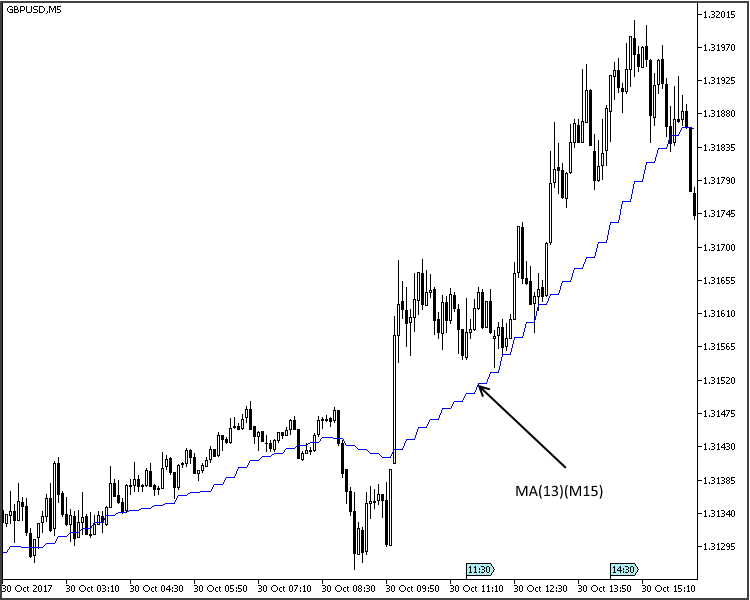Fig. 10. MTF indicator line

This method can be used for all types of linear indicators. The main disadvantage is obvious from the above screenshot: the steps. For MA such a behavior is not a problem and can even be useful, as this enables clear defining of support and resistance levels. But for oscillators in which we use patterns, this behavior hinders identification and drawing of such patterns. Moreover, the solution is unacceptable for WPR, CCI and similar indicators, as the line appearance changes beyond recognition.

To solve this problem, the last bar should be calculated taking into account the weighting factors. Let's add the 'Interpolate' global variable, which allows using both solutions.

```input bool               Interpolate     = true;
//--- main cycle
for(int i=limit;i>=0 && !IsStopped();i--)
{
int n;
datetime t=time[i];
ExtMA[i]=_CopyBuffer(MA_Handle,t);
if(!Interpolate) continue;
//---
datetime times= _iTime(t);
for(n = 1; i+n<rates_total && time[i+n]>= times; n++) continue;
double factor=1.0/n;
for(int k=1; k<n; k++)
ExtMA[i+k]=k*factor*ExtMA[i+n]+(1.0-k*factor)*ExtMA[i];
}
//---
```

In this variant we need to add the _iTime function, which will determine the bar number based in the opening time, for the current chart.

```//+------------------------------------------------------------------+
datetime _iTime(datetime start_time)
{
if(start_time < 0) return(-1);
datetime Arr[];
if(CopyTime(NULL,_tf, start_time, 1, Arr)>0)
return(Arr);
else return(-1);
}
//+------------------------------------------------------------------+
```

This looks like a normal line now.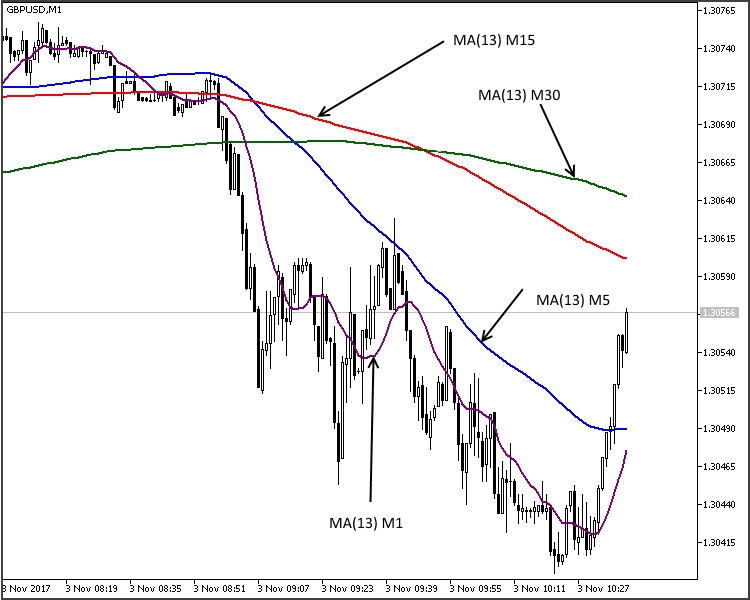Fig. 11. MTF indicator line with _iTime

Though development of such complex and resource intensive systems may seem inappropriate, they have their disadvantages and even can be indispensable. If classical averaging is used (MA, Alligator etc.), calculation period increase may lead to some delay compared to the MTF version. This is especially noticeable with small periods.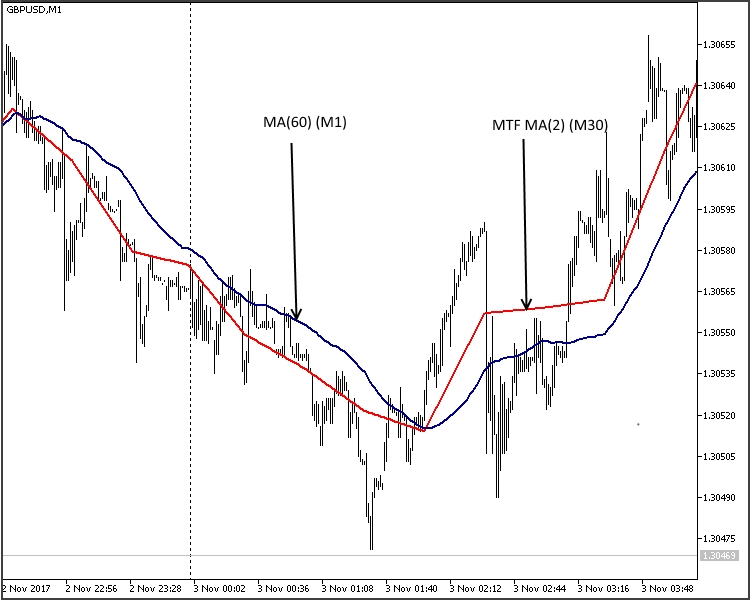Fig. 12. Lagging in the MTF MA indicator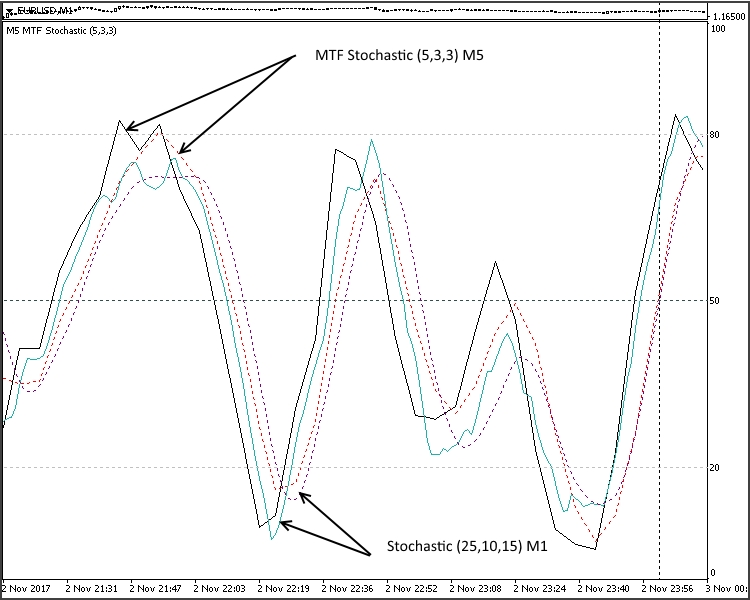Fig. 13. Lagging in the MTF Stochastic indicator

For simple indicators, such as MAs and Alligator, this may not be so significant. But for complex systems consisting of two or more MAs, such MACD, AO, etc. this can be significant. Moreover, there is no possibility to change the averaging period for AO or AC. As for indicators with a non-smoothing line (WPR, CCI, etc.) a trivial increase of the calculation period cannot provide a satisfactory result as they are very noisy.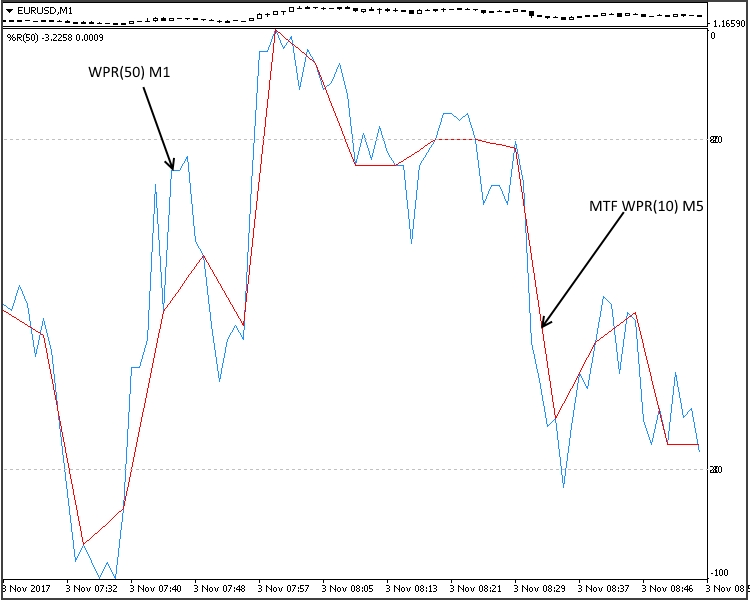Fig. 14. MTF indicator WRP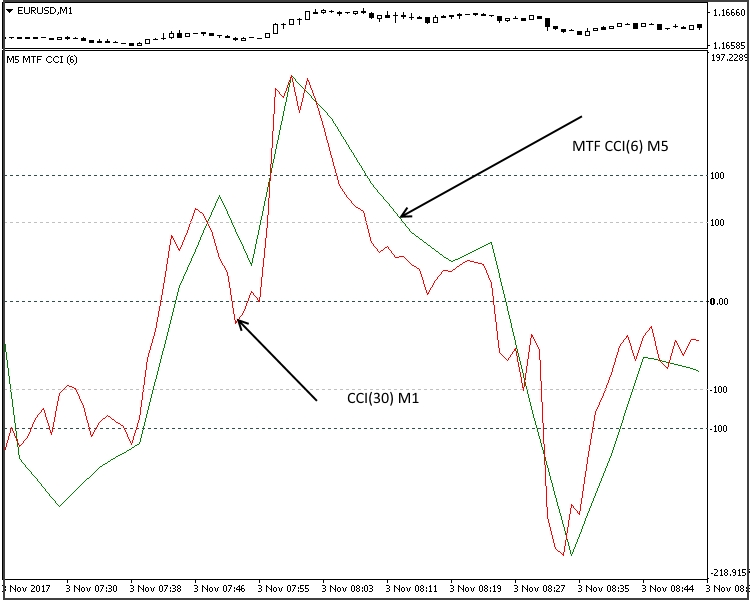Fig. 15. MTF indicaor CCI

Figures 14-15 show that they can be successfully used for smoothing, when this possibility is not provided for in the algorithm.

In addition to its direct function, this type can compensate for the shortcomings of the visual testing mode in MetaTrader 5 Strategy Tester. When creating MTF Expert Advisors for trading or analyzing the effectiveness of this type of strategy, we cannot simultaneously observe the position of indicators from different TFs on the screen. After testing we receive a set of tabs, depending on the number of periods used. Let us view the example of the "Three Elder screens" strategy from the article MQL5 Cookbook: Developing a Framework for a Trading System Based on the Triple Screen Strategy by Anatoli Kazharski. The idea of the strategy is as follows: the first timeframe is the largest one, such as weekly, daily or 4-hour. It is used to determine the main trend. The second timeframe differs from the first one by 1 or 2 orders. It is used to determine correction. The third timeframe differs by one more order. It is used to determine the optimal entry point.

In the first screen, which is usually M30-W1, launch MACD (12,26,1) and EMA with a period of 13. In the second screen, М5-D1, launch Stochastic Oscillator (5,3,3). The third screen from M1 to H4 is used for placing Stop orders in the main trend direction.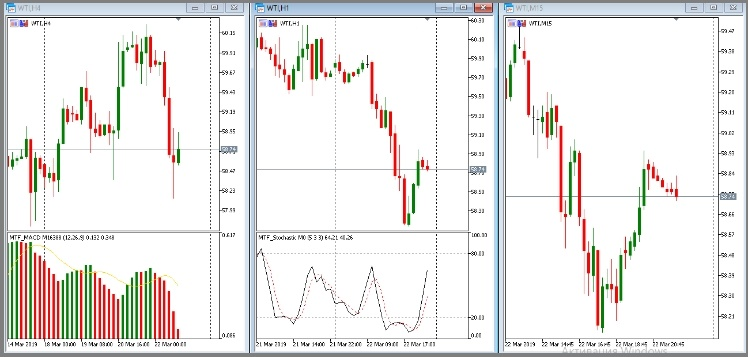Fig. 16. Elder's Three screens

The article author did not stick to this variant, but the concept of "Three screens" was preserved. What we see during the testing process and after it: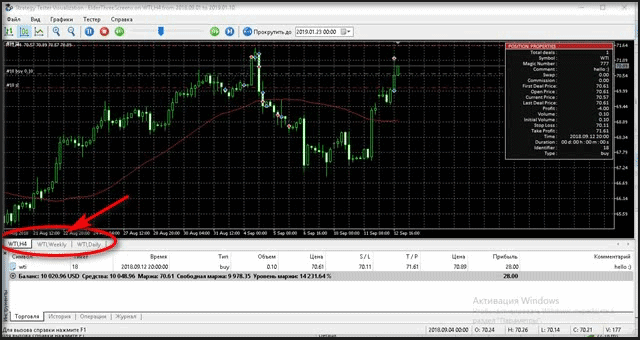Fig. 17. Testing of Elder's Three screen strategy

This variant does not allow us to properly analyze the operation of the expert Advisor (strategy).
Let's create our own EA version using our tools. It will be close to the classical strategy version. Creation of Expert Advisors based on the above indicators is very similar to classical EAs. Here is the main code.

```#define EXPERT_MAGIC 1234502
//---
#include <Trade\Trade.mqh>
#include <Trade\SymbolInfo.mqh>
#include <Trade\PositionInfo.mqh>
#include <Trade\AccountInfo.mqh>
#include <Trade\OrderInfo.mqh>
//+------------------------------------------------------------------+
enum compTF
{
A, //m1-m5-m15
B, //m5-m15-h1
C, //m15-h1-h4
E  //h1-h4-d1
};
//+------------------------------------------------------------------+
//|                                                                  |
//+------------------------------------------------------------------+
input string s0="//--- input parameters Lots+Trailing ---//";
input double InpLots          =0.1; // Lots
input int    InpTakeProfit    =150; // Take Profit (in pips)
input int    InpStopLoss      =60;  // StopLoss (in pips)
input int    InpLevel_S       =20;  // Level Signal
input compTF InpTFreg         =2;
input string s1="//--- input parameters MA ---//";
input int                Signal_MA_PeriodMA      =21;                             // Period of averaging
input string s2="//--- input parameters MACD ---//";
input int                Signal_MACD_PeriodFast  =12;                             // Period of fast EMA
input int                Signal_MACD_PeriodSlow  =26;                             // Period of slow EMA
input string s3="//--- input parameters Stochastic ---//";
input int                Signal_Stoch_PeriodK    =5;                              //  K-period
input int                Signal_Stoch_PeriodD    =3;                              //  D-period
input string s4="//--- input parameters Trailing ---//";
//--- inputs for trailing
input int    InpTrailingStop  =25;  // Trailing Stop Level (in pips)
input int    InpOffset        =5;   // Distance from the price (in pips)
//---
int ExtTimeOut=10; // time interval between trading operations in seconds
int barsCalculated=3;
datetime t=0;
datetime time[];
//+------------------------------------------------------------------+
//| AC Sample expert class                                           |
//+------------------------------------------------------------------+
class CSampleExpert
{
protected:
double            m_adjusted_point;             // 3- or 5-digit value
CTrade            m_trade;                      // trading object
CSymbolInfo       m_symbol;                     // symbol information
CPositionInfo     m_position;                   // trading position
CAccountInfo      m_account;                    // account information
COrderInfo        m_order;                      // order information
//--- indicators
ENUM_TIMEFRAMES   mas_tf;                   // array of timeframes
int               handle_MACD;                 // MACD indicator handle
int               handle_MA;                   // MA indicator handle
int               handle_Stochastic;           // Stochastic indicator handle
//--- indicator buffers
double            macd_buff[];           // MACD indicator main buffer
double            ma_buff[];             // MA indicator main buffer
double            stoch_buff[];          // Stochastic indicator main buffer
//---
double            close[];
double            open[];
double            low[];
double            high[];
//--- indicator data to process
double            macd_ind_0;
double            macd_ind_1;
double            ma_ind;
double            stoch_ind_0;
double            stoch_ind_1;
int               level_stoch;
//--- trailing stop data to process
double            m_traling_stop;
double            m_take_profit;
double            m_stop_losse;
public:
CSampleExpert(void);
~CSampleExpert(void);
bool              Init(void);
void              Deinit(void);
bool              Processing(void);

protected:
bool              InitCheckParameters(const int digits_adjust);
bool              Copy(void);              //
bool              InitIndicators(void);
bool              LongModified(void);
bool              ShortModified(void);
bool              LongOpened(void);          // check Long condition
bool              ShortOpened(void);         // check Short condition
bool              OpenSellStop(void);        // place the SELLSTOP order
bool              OpenBuyStop(void);         // place the BUYSTOP order
bool              OrderModifySellStop(void); // modify the SELLSTOP order
bool              OrderModifyBuyStop(void);  // modify the BUYSTOP order
bool              DellSellStop(void);        // delete the SELLSTOP order
bool              DellBuyStop(void);         // delete the BUYSTOP order
};
//--- global expert
CSampleExpert ExtExpert;
//+------------------------------------------------------------------+
//| Constructor                                                      |
//+------------------------------------------------------------------+
CSampleExpert::CSampleExpert(void) : m_adjusted_point(0),
handle_MACD(INVALID_HANDLE),
handle_Stochastic(INVALID_HANDLE),
handle_MA(INVALID_HANDLE),
macd_ind_0(0),
macd_ind_1(0),
stoch_ind_0(0),
stoch_ind_1(0),
ma_ind(0),
m_traling_stop(0),
m_take_profit(0)
{
ArraySetAsSeries(macd_buff,true);
}
//+------------------------------------------------------------------+
//| Destructor                                                       |
//+------------------------------------------------------------------+
CSampleExpert::~CSampleExpert(void)
{
}
//+------------------------------------------------------------------+
//| Initialization and verification of input parameters              |
//+------------------------------------------------------------------+
bool CSampleExpert::Init(void)
{
//--- initializing general information
m_symbol.Name(Symbol());                  // symbol
m_trade.SetExpertMagicNumber(EXPERT_MAGIC); // magic
m_trade.SetMarginMode();
m_trade.SetTypeFillingBySymbol(Symbol());
//--- tuning for 3 or 5 digits
int digits_adjust=1;
if(m_symbol.Digits()==3 || m_symbol.Digits()==5)
digits_adjust=10;
m_adjusted_point=m_symbol.Point()*digits_adjust;
//--- setting the default deviation for trading
m_traling_stop    =InpTrailingStop*m_adjusted_point;
m_take_profit     =InpTakeProfit*m_adjusted_point;
m_stop_losse      =InpStopLoss*m_adjusted_point;
//--- set default deviation for trading in adjusted points
m_trade.SetDeviationInPoints(3*digits_adjust);
//---
int x=InpTFreg;
switch(x)
{
case 0: {mas_tf=PERIOD_M1;mas_tf=PERIOD_M5;mas_tf=PERIOD_M15;}
break;
case 1: {mas_tf=PERIOD_M5;mas_tf=PERIOD_M15;mas_tf=PERIOD_H1;}
break;
case 2: {mas_tf=PERIOD_M15;mas_tf=PERIOD_H1;mas_tf=PERIOD_H4;}
break;
case 3: {mas_tf=PERIOD_H1;mas_tf=PERIOD_H4;mas_tf=PERIOD_D1;}
break;
}
//---
if(!InitCheckParameters(digits_adjust))
return(false);
if(!InitIndicators())
return(false);
//--- result
return(true);
}
//+------------------------------------------------------------------+
//| Verification of input parameters                                 |
//+------------------------------------------------------------------+
bool CSampleExpert::InitCheckParameters(const int digits_adjust)
{
//--- checking source data
if(InpTakeProfit*digits_adjust<m_symbol.StopsLevel())
{
printf("Take Profit must be greater than %d",m_symbol.StopsLevel());
return(false);
}
if(InpTrailingStop*digits_adjust<m_symbol.StopsLevel())
{
printf("Trailing Stop must be greater than %d",m_symbol.StopsLevel());
return(false);
}
//--- checking the lot value
if(InpLots<m_symbol.LotsMin() || InpLots>m_symbol.LotsMax())
{
printf("Lots must be in the range between %f and %f",m_symbol.LotsMin(),m_symbol.LotsMax());
return(false);
}
if(MathAbs(InpLots/m_symbol.LotsStep()-MathRound(InpLots/m_symbol.LotsStep()))>1.0E-10)
{
printf("The amount does not correspond to the lot step %f",m_symbol.LotsStep());
return(false);
}
//--- warning
if(InpTakeProfit<=InpTrailingStop)
printf("Warning: Trailing Stop must be less than Take Profit");
//--- result
return(true);
}
//+------------------------------------------------------------------+
//| Initialization of indicators                                     |
//+------------------------------------------------------------------+
bool CSampleExpert::InitIndicators(void)
{
//--- create the MACD indicator
if(handle_MACD==INVALID_HANDLE)
{
//---
handle_MACD=iCustom(NULL,0,"MTF\\Oscillators\\MTF_MACD",mas_tf,Signal_MACD_PeriodFast,Signal_MACD_PeriodSlow);
//---
if(handle_MACD==INVALID_HANDLE)
{
printf("Error occurred while creating MACD");
return(false);
}
}
//--- create the MA indicator
if(handle_MA==INVALID_HANDLE)
{
//---
handle_MA=iCustom(NULL,0,"MTF\\Trend\\MA_MultiTF",mas_tf,Signal_MA_PeriodMA,0,MODE_EMA,PRICE_CLOSE);
//---
if(handle_MA==INVALID_HANDLE)
{
printf("Error occurred while creating MA");
return(false);
}
}
//--- create the Stochastic indicator
if(handle_Stochastic==INVALID_HANDLE)
{
//---
handle_Stochastic=iCustom(NULL,0,"MTF\\Oscillators\\MTF_Stochastic",mas_tf,Signal_Stoch_PeriodK,Signal_Stoch_PeriodD);
//---
if(handle_Stochastic==INVALID_HANDLE)
{
printf("Error occurred while creating Stochastic");
return(false);
}
}
//--- result
return(true);
}
//+------------------------------------------------------------------+
//|          Modifying the long position                             |
//+------------------------------------------------------------------+
bool CSampleExpert::LongModified(void)
{
bool res=false;
//--- checking the trailing stop
if(InpTrailingStop>0)
{
if(m_symbol.Bid()-m_position.PriceOpen()>m_adjusted_point*InpTrailingStop)
{
double sl=NormalizeDouble(m_symbol.Bid()-m_traling_stop,m_symbol.Digits());
double tp=m_position.TakeProfit();
if(m_position.StopLoss()<sl || m_position.StopLoss()==0.0)
{
//--- modifying the position
if(m_trade.PositionModify(Symbol(),sl,tp))
printf("Long position by %s to be modified",Symbol());
else
{
printf("Error modifying position by %s : '%s'",Symbol(),m_trade.ResultComment());
printf("Modify parameters : SL=%f,TP=%f",sl,tp);
}
//--- was modified and must exit the Expert Advisor
res=true;
}
}
}
//--- result
return(res);
}
//+------------------------------------------------------------------+
//|                    Modifying the short position                  |
//+------------------------------------------------------------------+
bool CSampleExpert::ShortModified(void)
{
bool   res=false;
//--- checking the trailing stop
if(InpTrailingStop>0)
{
if((m_position.PriceOpen()-m_symbol.Ask())>(m_adjusted_point*InpTrailingStop))
{
double sl=NormalizeDouble(m_symbol.Ask()+m_traling_stop,m_symbol.Digits());
double tp=m_position.TakeProfit();
if(m_position.StopLoss()>sl || m_position.StopLoss()==0.0)
{
//--- modifying the position
if(m_trade.PositionModify(Symbol(),sl,tp))
printf("Short position by %s to be modified",Symbol());
else
{
printf("Error modifying position by %s : '%s'",Symbol(),m_trade.ResultComment());
printf("Modify parameters : SL=%f,TP=%f",sl,tp);
}
//--- was modified and must exit the Expert Advisor
res=true;
}
}
}
//--- result
return(res);
}
//+------------------------------------------------------------------+
//| Checking conditions for opening a long position                  |
//+------------------------------------------------------------------+
bool CSampleExpert::LongOpened(void)
{
bool res=false;
level_stoch=InpLevel_S;
//--- checking the possibility to open a long (BUY) position
if(stoch_ind_1<level_stoch && stoch_ind_0>level_stoch && macd_ind_1>macd_ind_0 && ma_ind<close && ma_ind<open)//&& ma_ind<close && ma_ind<open
{
//--- exit the EA
res=true;
}
//--- result
return(res);
}
//+------------------------------------------------------------------+
//|         Opening a Buy Stop order                                 |
//+------------------------------------------------------------------+
bool CSampleExpert::OpenBuyStop(void)
{
bool res=false;
double tp=0,sl=0;
//---
if(LongOpened())
{
res=true;
//--- declare and initialize the trade request and result
MqlTradeRequest request={0};
MqlTradeResult  result={0};
//--- pending order placing parameters
request.action   =TRADE_ACTION_PENDING;                             // trading operation type
request.symbol   =Symbol();                                         // symbol
request.deviation=5;                                                // allowed deviation from the price
request.volume   =InpLots;                                          // volume in lots
request.magic    =EXPERT_MAGIC;                                     // order MagicNumber
double offset=InpOffset;                                            // point distance from the current price to place the order
double price;                                                       // order trigger price
double point=SymbolInfoDouble(_Symbol,SYMBOL_POINT);                // point size
int digits=(int)SymbolInfoInteger(_Symbol,SYMBOL_DIGITS);           // number of decimal places (digits)
//--- operation type
request.type=ORDER_TYPE_BUY_STOP;                                   // order type
price=high+offset*m_adjusted_point;                              // open price
request.price=NormalizeDouble(price,digits);                        // normalized open price
tp=price+m_take_profit;
sl=price-m_stop_losse;
request.sl       =NormalizeDouble(sl,_Digits);                       // add the new Stop Loss value to the structure
request.tp       =NormalizeDouble(tp,_Digits);                       // add the new Take Profit value to the structure
//--- sending a request
if(!OrderSend(request,result))
{res=false;printf("OrderSend error %d",GetLastError());}           // if unable to send the request, output the error code
//--- information about the operation
printf("retcode=%u  deal=%I64u  order=%I64u",result.retcode,result.deal,result.order);
//--- exit the EA
}
//--- result
return(res);
}
//+------------------------------------------------------------------+
//| Checking conditions for opening a short position                 |
//+------------------------------------------------------------------+
bool CSampleExpert::ShortOpened(void)
{
bool res=false;
level_stoch=100-InpLevel_S;
//--- checking the possibility of a short position (SELL)
if(stoch_ind_1>level_stoch && stoch_ind_0<level_stoch && macd_ind_1<macd_ind_0 && ma_ind>close && ma_ind>open)
{
//--- exit the EA
res=true;
}
//--- result
return(res);
}
//+------------------------------------------------------------------+
//|         Opening a Sell Stop order                                 |
//+------------------------------------------------------------------+
bool CSampleExpert::OpenSellStop(void)
{
bool res=false;
double tp=0,sl=0;
//---
if(ShortOpened())
{
res=true;
//--- declare and initialize the trade request and result
MqlTradeRequest request={0};
MqlTradeResult  result={0};
//--- pending order placing parameters
request.action   =TRADE_ACTION_PENDING;                             // trading operation type
request.symbol   =Symbol();                                         // symbol
request.deviation=5;                                                // allowed deviation from the price
request.volume=InpLots;                                             // volume in lots
request.magic=EXPERT_MAGIC;                                         // order MagicNumber
int offset=InpOffset;                                                // point distance from the current price to place the order
double price;                                                       // order trigger price
int digits=(int)SymbolInfoInteger(_Symbol,SYMBOL_DIGITS);           // number of decimal places (digits)
request.type=ORDER_TYPE_SELL_STOP;                                  // order type
price=low-offset*m_adjusted_point;                                          // open price
request.price=NormalizeDouble(price,digits);                        // normalized open price
tp=price-m_take_profit;
sl=price+m_stop_losse;
request.sl       =NormalizeDouble(sl,_Digits);                       // add the new Stop Loss value to the structure
request.tp       =NormalizeDouble(tp,_Digits);                       // add the new Take Profit value to the structure
//--- sending a request
if(!OrderSend(request,result))
{res=false;printf("OrderSend error %d",GetLastError());}     // if unable to send the request, output the error code
//--- information about the operation
printf("retcode=%u  deal=%I64u  order=%I64u",result.retcode,result.deal,result.order);
//--- exit the EA
}
//--- result
return(res);
}
//+------------------------------------------------------------------+
//| The main function returns true if any of the positions           |
//| is being processed                                               |
//+------------------------------------------------------------------+
bool CSampleExpert::Processing(void)
{
//   MqlDateTime dt;
//--- Update frequency
if(!m_symbol.RefreshRates())
return(false);
//--- Update indicators
if(BarsCalculated(handle_Stochastic)<barsCalculated)
return(false);
if(CopyBuffer(handle_Stochastic,0,0,barsCalculated,stoch_buff)!=barsCalculated)
return(false);
//---
if(BarsCalculated(handle_MACD)<barsCalculated)
return(false);
if(CopyBuffer(handle_MACD,0,0,barsCalculated,macd_buff)!=barsCalculated)
return(false);
//---
if(BarsCalculated(handle_MA)<barsCalculated)
return(false);
if(CopyBuffer(handle_MA,0,0,barsCalculated,ma_buff)!=barsCalculated)
return(false);
//---
if(!Copy())return(false);
//--- to simplify programming and provide a faster access
//--- data are located in internal variables
macd_ind_0   = macd_buff;
macd_ind_1   = macd_buff;
ma_ind       = ma_buff;
stoch_ind_0  = stoch_buff;
stoch_ind_1  = stoch_buff;

//--- it is important to correctly exit it ...
//--- First check if the position exists - try to select it
bool bord=false,sord=false;
ulong ticket;
//+--- There are open positions and trailing stop is enabled --------+
if(m_position.Select(Symbol()) && PositionsTotal()>0 && m_traling_stop!=0)
{
if(m_position.PositionType()==POSITION_TYPE_BUY)
{
bord=true;
//--- modifying the long position
if(LongModified())
return(true);
}
else
{
sord=true;
//--- modifying the short position
if(ShortModified())
return(true);
}
}
//+--------- trading STOP orders ------------------------------------+
// In this cycle, check all placed pending orders one by one
for(int i=0;i<OrdersTotal();i++)
{
// select each of orders and getting its ticket
ticket=OrderGetTicket(i);
// handling Buy Stop orders
if(m_order.OrderType()==ORDER_TYPE_BUY_STOP)
{
// setting the flag indicating that there is a Buy Stop order
bord=true;
//--- It is important to enter the market correctly, move the order if necessary
if(bord)OrderModifyBuyStop(); // modify the BUYSTOP order
}
// handling Sell Stop orders
if(m_order.OrderType()==ORDER_TYPE_SELL_STOP)
{
// setting the flag indicating that there is a Sell Stop order
sord=true;
//--- It is important to enter the market correctly, move the order if necessary
if(sord)OrderModifySellStop(); // modify the SELLSTOP order
}
}
//--- If there are no orders, place --------------------------------+
if(!sord)if(OpenSellStop()){sord=true;return(true);}
if(!bord)if(OpenBuyStop()){bord=true;return(true);}
//--- exit without position handling
return(false);
}
//+------------------------------------------------------------------+
//|Changing parameters of the earlier placed Sell Stop trading order |
//+------------------------------------------------------------------+
bool CSampleExpert::OrderModifySellStop(void)
{
bool res=true;
ulong ticket;
double tp=0,sl=0;
double offset=InpOffset;                                            // point distance from the current price to place the order
double price;                                                       // order trigger price
int digits=(int)SymbolInfoInteger(_Symbol,SYMBOL_DIGITS);           // number of decimal places (digits)
// declare and initialize the trade request and result
MqlTradeRequest request={0};
MqlTradeResult  result={0};
int total=OrdersTotal(); // number of pending orders
//--- iterate over all placed pending orders
for(int i=total-1; i>=0; i--)
{
// select each of orders and getting its ticket
ticket=OrderGetTicket(i);
// handling Sell Stop orders
if(m_order.OrderType()==ORDER_TYPE_SELL_STOP)
{
ulong  magic=OrderGetInteger(ORDER_MAGIC);               // order MagicNumber
//--- if MagicNumber matches
if(magic==EXPERT_MAGIC)
{
price=low-offset*m_adjusted_point;                         // open price
if(price>m_order.PriceOpen()) // check the conditions
if(low>low) // check the conditions
{
request.action=TRADE_ACTION_MODIFY;                           // trading operation type
request.order = OrderGetTicket(i);                            // order ticket
request.symbol   =Symbol();                                   // symbol
request.deviation=InpOffset;                                  // allowed deviation from the price
price=low-offset*m_adjusted_point;                         // open price
request.price=NormalizeDouble(price,digits);                  // normalized open price
tp=price-m_take_profit;
sl=price+m_stop_losse;
request.sl       =NormalizeDouble(sl,_Digits);                // add the new Stop Loss value to the structure
request.tp       =NormalizeDouble(tp,_Digits);                // add the new Take Profit value to the structure
//--- sending a request
if(!OrderSend(request,result))
{
// setting the flag indicating that the Sell Stop order has not been deleted
res=true; printf("OrderSend error %d",GetLastError());
}  // if unable to send the request, output the error code
//--- information about the operation
printf("retcode=%u  deal=%I64u  order=%I64u",result.retcode,result.deal,result.order);
}
}
}
}
return(res);
}
//+------------------------------------------------------------------+
//|Changing parameters of the earlier placed Buy Stop trading order  |
//+------------------------------------------------------------------+
bool CSampleExpert::OrderModifyBuyStop(void)
{
bool res=true;
ulong ticket;
double tp=0,sl=0;
double offset=InpOffset;                                            // point distance from the current price to place the order
double price;                                                       // order trigger price
int digits=(int)SymbolInfoInteger(_Symbol,SYMBOL_DIGITS);           // number of decimal places (digits)
//--- declare and initialize the trade request and result
MqlTradeRequest request={0};
MqlTradeResult  result={0};
int total=OrdersTotal(); // number of pending orders
//--- iterate over all placed pending orders
for(int i=total-1; i>=0; i--)
{
// select each of orders and getting its ticket
ticket=OrderGetTicket(i);
// handling Buy Stop orders
if(m_order.OrderType()==ORDER_TYPE_BUY_STOP)
{
ulong  magic=OrderGetInteger(ORDER_MAGIC);               // order MagicNumber
//--- if MagicNumber matches
if(magic==EXPERT_MAGIC)
{
price=high+offset*m_adjusted_point;                        // open price
if(price<m_order.PriceOpen()) // check the conditions
{
request.action=TRADE_ACTION_MODIFY;                           // trading operation type
request.symbol   =Symbol();                                   // symbol
request.action=TRADE_ACTION_MODIFY;                           // trading operation type
request.order = OrderGetTicket(i);                            // order ticket
request.symbol   =Symbol();                                   // symbol
request.deviation=InpOffset;                                  // allowed deviation from the price
//--- set the price level, take profit and stop loss

request.price=NormalizeDouble(price,digits);                  // normalized open price
tp=price+m_take_profit;
sl=price-m_stop_losse;
request.sl       =NormalizeDouble(sl,_Digits);                       // add the new Stop Loss value to the structure
request.tp       =NormalizeDouble(tp,_Digits);                       // add the new Take Profit value to the structure
//--- sending a request
if(!OrderSend(request,result))
{
// setting the flag indicating that the Buy Stop order has not been deleted
res=true;
printf("OrderSend error %d",GetLastError());
}  // if unable to send the request, output the error code
//--- information about the operation
printf("retcode=%u  deal=%I64u  order=%I64u",result.retcode,result.deal,result.order);
}
}
}
}
return(res);
}
//+------------------------------------------------------------------+
//| Expert initialization function                                   |
//+------------------------------------------------------------------+
int OnInit(void)
{
//--- creating all required object
if(!ExtExpert.Init())
return(INIT_FAILED);
//--- ok
return(INIT_SUCCEEDED);
}
//+------------------------------------------------------------------+
//| Tick processing function                                         |
//+------------------------------------------------------------------+
void OnTick(void)
{
static datetime limit_time=0; // the last trade processing time + timeout
//--- do not handle is timeout
if(TimeCurrent()>=limit_time)
{
//--- checking data
if(Bars(Symbol(),Period())>barsCalculated)
{
//--- checking the limit time in terms of timeout in seconds, if processed
if(ExtExpert.Processing())
limit_time=TimeCurrent()+ExtTimeOut;
}
}
}
//+------------------------------------------------------------------+
//|                                                                  |
//+------------------------------------------------------------------+
bool CSampleExpert::Copy(void)
{
//--- amount to copy
int copied=3;
//---
ArrayResize(high,copied);
ArrayResize(low,copied);
ArrayResize(close,copied);
ArrayResize(open,copied);
//+------- Setting the array indexing direction ---------------------+
ArraySetAsSeries(high,true);
ArraySetAsSeries(low,true);
ArraySetAsSeries(close,true);
ArraySetAsSeries(open,true);
//--- copying bar prices
if(CopyHigh(NULL,mas_tf,0,copied,high)<0)
{printf("No copied High",GetLastError());return(false);}
//---
if(CopyLow(NULL,mas_tf,0,copied,low)<0)
{printf("No copied Low",GetLastError());return(false);}
//---
if(CopyClose(NULL,mas_tf,0,copied,close)<0)
{printf("No copied Close",GetLastError());return(false);}
//---
if(CopyOpen(NULL,mas_tf,0,copied,open)<0)
{printf("No copied Open",GetLastError());return(false);}
//---
return(true);
}
//+------------------------------------------------------------------+

```

The use of this EA is much more convenient: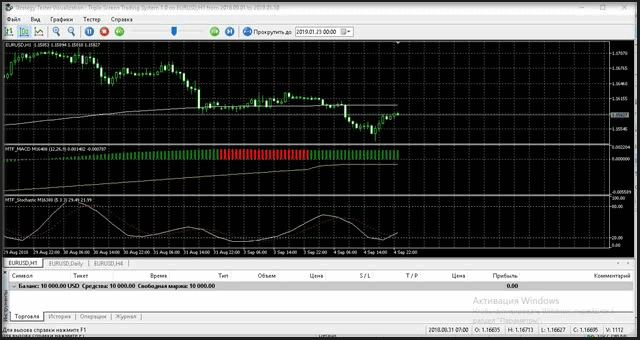Fig. 18. EA testing with the implemented MTF tools

Now in addition to viewing the operation of the Expert Advisor, we can also analyze its possible disadvantages and thus benefit from the wide possibilities of the tester.

### Conclusion

Although the authors of the MQL5 software modules do not provide for the direct possibility to create such algorithms, these indicators can be very useful. In some cases, they enable the simultaneous market state analysis at different TFs, improvement of the strategy tester efficiency and the improvement of the smoothing. The presented code examples may have disadvantages, so there are a lot of opportunities for further development of the MTF indicator idea using the MQL5 programming language.

Attachments

Name Type Location
MA_MultiPeriod Trend MQL5\Indicators\MA_MultiPeriod.mq5
MA_MultiTF Trend MQL5\Indicators\MTF\Trend\MA_MultiTF.mq5
MTF_BB Trend MQL5\Indicators\MTF\Trend\MTF_BB.mq5
MTF_Envelopes Trend MQL5\Indicators\MTF\Trend\MTF_Envelopes.mq5
MTF_ParabolicSAR Trend MQL5\Indicators\MTF\Trend\MTF_ParabolicSAR.mq5
MTF_StdDev Trend MQL5\Indicators\MTF\Trend\MTF_StdDev.mq5
MTF_CCI Oscillators MQL5\Indicators\MTF\Oscillators\MTF_CCI.mq5
MTF_Force_Index Oscillators MQL5\Indicators\MTF\Oscillators\MTF_Force_Index.mq5
MTF_MACD Oscillators MQL5\Indicators\MTF\Oscillators\MTF_MACD.mq5
MTF_Momentum Oscillators MQL5\Indicators\MTF\Oscillators\MTF_Momentum.mq5
MTF_RSI Oscillators MQL5\Indicators\MTF\Oscillators\MTF_RSI.mq5
MTF_RVI Oscillators MQL5\Indicators\MTF\Oscillators\MTF_RVI.mq5
MTF_Stochastic Oscillators MQL5\Indicators\MTF\Oscillators\MTF_Stochastic.mq5
MTF_WPR  Oscillators  MQL5\Indicators\MTF\Oscillators\MTF_WPR.mq5
Triple Screen Trading System 1.0  Expert Advisor

Translated from Russian by MetaQuotes Software Corp.
Original article: https://www.mql5.com/ru/articles/2837

Attached files |
MTF.zip (147.76 KB)
Last comments | Go to discussion (1)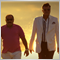| 14 May 2019 at 03:42

I try to test EA but it don't buy and sell , and gives error.

Not all data of Handle is calculated (-1bars ). Error4806

Not all data of ExtHandle1 is calculated (-1bars ). Error4806

Not all data of Handle is calculated (-1bars ). Error4806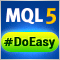Library for easy and quick development of MetaTrader programs (part III). Collection of market orders and positions, search and sorting

In the first part, we started creating a large cross-platform library simplifying the development of programs for MetaTrader 5 and MetaTrader 4 platforms. Further on, we implemented the collection of history orders and deals. Our next step is creating a class for a convenient selection and sorting of orders, deals and positions in collection lists. We are going to implement the base library object called Engine and add collection of market orders and positions to the library.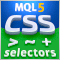Extracting structured data from HTML pages using CSS selectors

The article provides a description of a universal method for analyzing and converting data from HTML documents based on CSS selectors. Trading reports, tester reports, your favorite economic calendars, public signals, account monitoring and additional online quote sources will become available straight from MQL.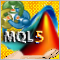Using MATLAB 2018 computational capabilities in MetaTrader 5

After the upgrade of the MATLAB package in 2015, it is necessary to consider a modern way of creating DLL libraries. The article uses a sample predictive indicator to illustrate the peculiarities of linking MetaTrader 5 and MATLAB using modern 64-bit versions of the platforms, which are utilized nowadays. With the entire sequence of connecting MATLAB considered, MQL5 developers will be able to create applications with advanced computational capabilities much faster, avoiding «pitfalls».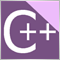A DLL for MQL5 in 10 Minutes (Part II): Creating with Visual Studio 2017

The original basic article has not lost its relevance and thus if you are interested in this topic, be sure to read the first article. However much time has passed since then, so the current Visual Studio 2017 features an updated interface. The MetaTrader 5 platform has also acquired new features. The article provides a description of dll project development stages, as well as DLL setup and interaction with MetaTrader 5 tools.